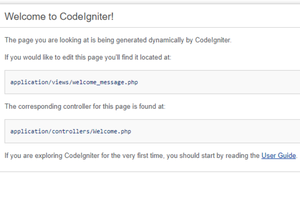GeeksforGeeks App
Open AppBrowser
Continue

# Describe a CodeIgniter ‘model’ in detail

In this article, we will discuss the model in CodeIgniter in detail?

At the first, let’s discuss the MVC structure in CodeIgniter. As we know over decades website has gone from simple HTML with CSS to complex application with hundreds of developers working on them

To make working on these complex applications simple we use different patterns to layout the project to make the code less complex and easier to work on it. The most popular pattern is MVC known as model, view, and controller.

The goal of the MVC pattern is to split the large application into specific sections such that all of them have their own purpose.

Explain how MVC works?

Suppose a user sends a request to the server to get the list of all courses. The server will send this request to the controller that handles the list of courses.

The controller then asks the model to return a list of courses available. The model would query the database for the list of all courses and then return the list back to the controller. If the response back from the model was successful then the controller would ask the view associated with courses to return a presentation of the list of courses.

The view would take the list of courses from the controller and render the list into HTML that could be used by the browser. The controller would then take that presentation and return it back to the user thus ending the request.

We can see from the example that the model handles all the data, the view handle all the presentation and the controller just tells the model and view what to do.

Model in CodeIgniter

As we have seen from the above example that model handles all the data. It mainly deals with the data. Suppose we want to interact with the database then the model would be responsible for that. The controller doesn’t interact with the database directly, the controller asks the model to return the data from the database. Then model would query the database and then return the requested data to the controller.

So model jobs are mainly:

• Implement the data logic to fetch the data from the database
• Respond to the controller when it asks for any data
• It stores, modify and delete the data from the database

It is basically a class in PHP that is responsible for interacting with the database part. Suppose we have a blog in which we insert, modify and delete the records then our model class would look something like this:

Syntax: The basic model would look like this:

```class Model_name extends CI_Model {
function __construct()
{
parent::__construct();
}
}```

Model_name is the name of your class. The name of your class must be starting with a capital letter. The name of the file must be the same as the name of the class.

Example:

## PHP

 `class` `My_blog_model ``extends` `CI_Model {` `    ``public` `\$title``;``    ``public` `\$content``;``    ``public` `\$date``;` `    ``public` `function` `get_last_5_entries() {``        ``\$query` `= ``\$this` `-> db -> get(``'entries'``, 5);``        ``return` `\$query` `-> result();``    ``}` `    ``public` `function` `insert_entry() {``        ``\$this` `-> title    = ``\$_POST``[``'title'``];``        ``\$this` `-> content  = ``\$_POST``[``'content'``];``        ``\$this` `-> ``date`     `= time();` `        ``\$this` `-> db -> insert(``'entries'``, ``\$this``);``    ``}` `    ``public` `function` `update_entry() {``        ``\$this` `-> title    = ``\$_POST``[``'title'``];``        ``\$this` `-> content  = ``\$_POST``[``'content'``];``        ``\$this` `-> ``date`     `= time();` `        ``\$this` `-> db -> update(``'entries'``, ``\$this``,``                              ``array``(``'id'` `=> ``\$_POST``[``'id'``]));``    ``}``}`

Once your CodeIgniter has been successfully installed and run then you will see the following screen.Loading a Model: When we use a model then we need to load our model inside the controller file. So that it could be called from the controller methods.

We can load our model by following way:

`\$this->load->model('Name_Of_The_Model');  `

Suppose if our model file is located inside a sub-directory then we need to mention the full path. For example, if our model file is present inside the GFG folder then we need to add:

`\$this->load->model('GFG/Name_Of_The_Model');  `

Once we have loaded our model file then we can access the model methods using the object that we have created

```\$this->load->model('my_model');
\$this->my_model->method();```

There are two ways to connect the model to the database:

• Auto-connect
• Manual connect

Auto Connect: To auto-connect, the model to the database adds the following code inside your autoload.php file.

`\$autoload['libraries'] = array('database);`

Manual connect: We need to add the following code to the page where the model is needed:

`\$this->load->database();  `

My Personal Notes arrow_drop_up
Related Tutorials